## Circuit Breaker Rating:

The ratings of a circuit breaker refer to the characteristic values that define the working conditions for which the circuit breaker is designed and built. Circuit breakers must be capable of carrying continuously the full load current, without excessive temperature rise, and should be capable of withstanding the electrodynamic forces. The circuit breaker should also be in a position to interrupt fault currents safely. The standard ratings of different classes of circuit breakers are given in various national and international standards. According to I.E.C. Specifications 56-1 (1954) and American Standards, an a.c. Circuit Breaker Rating are as follows:

1. Rated voltage, rated current.
2. Rated frequency.
3. Rated breaking capacities, symmetrical and asymmetrical.
4. Rated making capacities.
5. Rated short time current or rated maximum duration of short circuit.
6. Rated operating duty.

1. Rated Voltage: During normal operating conditions the voltage at any point of the power system is not constant. Due to this the manufacturer guarantees perfect operation of the circuit breaker at rated maximum voltage, which as a rule is higher than rated nominal voltage.

The rated maximum voltage of a circuit breaker is the highest rats voltage, above nominal system voltage, for which the circuit breaker is designed and is the upper limit for operation.

Rated current: The rated current of a circuit breaker is the designated limit of current in rats amperes which it shall be capable of carrying continuously without exceeding the limit of observable temperature rise.

The temperature rise of each of the various parts of the circuit breaker when tested to demonstrate rated continuous current, shall not exceed the limit given for that part as indicated in Table 14.1.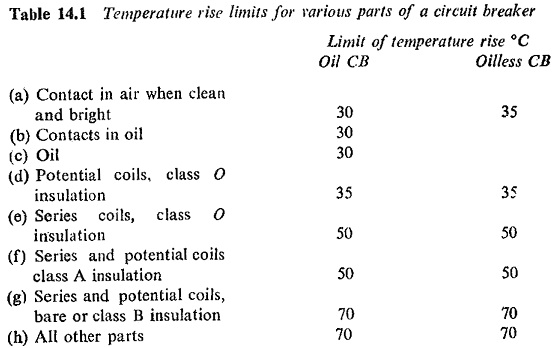For circuit breakers installed in enclosures, these temperature rise limits are based on the ambient temperature inside the enclosure, which should not exceed 40°C it the circuit breakers have copper to copper contacts, or 55°C if the circuit breakers have silver or other contacts.

Care should be taken to consider the possible increase of temperature rise of contacts in air due to oxidation of the contact surfaces. The breaker can retain its rating provided there is sufficient maintenance to keep the temperature rise within the specified limits.

For applications at altitudes higher than 1000 m the basic impulse insulation level (BIL) rated maximum voltage, and rated current shall be multiplied individually by the following correction factors given in Table 14.2.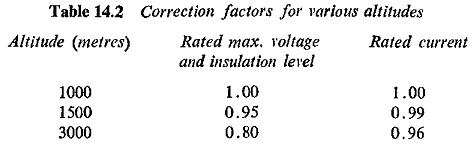Interpolated correction factors shall be used in determining correction factors for intermediate altitudes.

2. Rated Frequency: The rated frequency of the circuit breaker is the frequency at which it is designed to operate. Standard frequency is 50 Hz. Applications at other frequencies should receive special consideration.

Fated Breaking Capacities, Symmetrical and Asymmetrical: It can be seen from the shape of the short-circuit current Fig. (14.1) that the tins value of the current varies with time on account of the presence of d.c component of the current which decreases with time.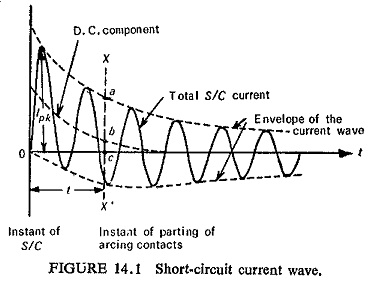After the instant of fault, the short circuit current starts decaying from a high initial value to a sustained value. In addition, owing to relaying time the circuit breaker starts to open its arcing contacts only some time later, after the initiation of short circuit. Therefore, the actual current interrupted by the circuit breaker is less than the initial value of short-circuit current.

The IEC definition of breaking current is as follows:

3. Breaking Current: The breaking current of a pole of a circuit breaker is the current in that pole at the instant of contact-separation. It is expressed by two values.

(a) The symmetrical breaking current: This is the rms value of a.c. component of the current in the pole at the instant of contact separation.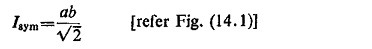(b) The asymmetrical breaking current: This is the rms value of the total current comprising the a c. and d.c. components of the current in that pole at the instant of contact separation.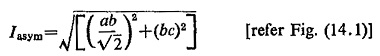Now according to these two values of breaking currents there are two corresponding values of breaking capacities. Conventionally the breaking capacity in MVA is equal to √3 times the rated voltage in KV times the rated breaking current in KA. However, the breaking capacities can now be defined as follows:

• The symmetrical breaking capacity, is the value of symmetrical breaking current which the circuit breaker is capable of breaking at a stated recovery voltage and a stated reference restriking voltage under prescribed
• The asymmetrical breaking capacity is the value of the asymmetrical breaking current which the circuit breaker is capable of breaking at a stated recovery voltage and a stated reference restriking voltage under prescribed conditions.

4. Rated Making Current Capacities: This value characterizes the capability of breakers to close its contacts against short-circuit currents.

Making currents: The making current of a circuit breaker, when closed on a short circuit, is the rms value of the total current (including both a.c. and d.c. components) which is measured from the envelope of the current wave at the time of its first major peak.

The making current may also be expressed in terms of instantaneous value of current in which case it is measured at the first major peak of the current wave. This value is known as designed peak making current.

Figure (14.1) shows the short-circuit current wave, where Ipk represents the peak making current.

The making capacity of a circuit breaker is the current that the circuit breaker is capable of making at stated voltage under prescribed conditions of use and behavior.

The rated making capacities of a Circuit Breaker Rating are those which correspond to rated voltage. The absence of any indication to the contrary on the name plate implies that each rated making capacity is of the value given by: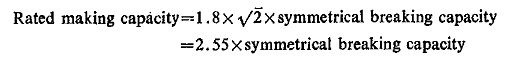Factor 1.8 is employed to account for the asymmetry present in the short-circuit current.

5. Rated Short Time Current: The short time current of a circuit breaker is the rms value of current that a Circuit Breaker Rating can carry in a fully closed position without damage, for the specified short time interval under prescribed conditions. It is normally expressed in terms of KA for a period of 1 sec or 4 sec, known as one-second rating and four-second rating, respectively. These ratings are based on thermal limitations.

No similar short time ratings are given for low-voltage breakers because such breakers are normally equipped with direct acting series overload trips.

6. Rated Operating Duty: The operating duty of a Circuit Breaker Rating consists of a prescribed number of unit operations at stated intervals.

According to IEC recommendations for rated operating duty for breakers which are not intended for auto-reclosure, there are two alternatives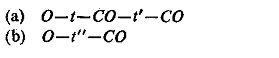Where

O = opening operation

C = closing operation

CO = closing followed by opening

t,t′,t″ = time intervals, t′ and t″ are expressed in minutes, t″ in seconds

Circuit breakers with auto-reclosures have the operating duty as follows: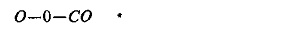0 is the dead time of the circuit breaker expressed in cycles.

The various components of operating time of circuit breaker are illustrated in Fig. (14.2).

Scroll to Top